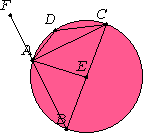# Proposition 31

In a circle the angle in the semicircle is right, that in a greater segment less than a right angle, and that in a less segment greater than a right angle; further the angle of the greater segment is greater than a right angle, and the angle of the less segment is less than a right angle.

Let ABCD be a circle, let BC be its diameter, and E its center. Join BA, AC, AD, and DC.

I say that the angle BAC in the semicircle BAC is right, the angle ABC in the segment ABC greater than the semicircle is less than a right angle, and the angle ADC in the segment ADC less than the semicircle is greater than a right angle.Join AE, and carry BA through to F.

I.5

Then, since BE equals EA, the angle ABE also equals the angle BAE. Again, since CE equals EA, the angle ACE also equals the angle CAE. Therefore the whole angle BAC equals the sum of the two angles ABC and ACB.

I.32

But the angle FAC exterior to the triangle ABC also equals the sum of the two angles ABC and ACB. Therefore the angle BAC also equals the angle FAC. Therefore each is right. Therefore the angle BAC in the semicircle BAC is right.

I.17

Next, since in the triangle ABC the sum of the two angles ABC and BAC is less than two right angles, and the angle BAC is a right angle, the angle ABC is less than a right angle. And it is the angle in the segment ABC greater than the semicircle.

III.22

Next, since ABCD is a quadrilateral in a circle, and the sum of the opposite angles of quadrilaterals in circles equals two right angles, while the angle ABC is less than a right angle, therefore the remaining angle ADC is greater than a right angle. And it is the angle in the segment ADC less than the semicircle.

I say further that the angle of the greater segment, namely that contained by the circumference ABC and the straight line AC, is greater than a right angle, and the angle of the less segment, namely that contained by the circumference ADC and the straight line AC, is less than a right angle.

This is at once manifest. For, since the angle contained by the straight lines BA and AC is right, the angle contained by the circumference ABC and the straight line AC is greater than a right angle.

Again, since the angle contained by the straight lines AC and AF is right, the angle contained by the straight line CA and the circumference ADC is less than a right angle.

Therefore in a circle the angle in the semicircle is right, that in a greater segment less than a right angle, and that in a less segment greater than a right angle; further the angle of the greater segment is greater than a right angle, and the angle of the less segment is less than a right angle.

Q.E.D.

## Guide

The part of this proposition which says that an angle inscribed in a semicircle is a right angle is often called Thale’s theorem.

This proposition is used in III.32 and in each of the rest of the geometry books, namely, Books IV, VI, XI, XII, XIII. It is also used in Book X.